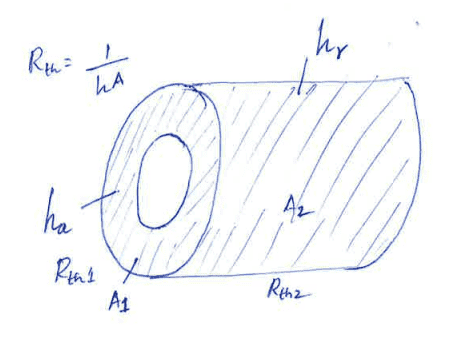# Need explanation on Chracteristic Length in heat convection

• thiru muruga

#### thiru muruga

Hi,

I am working on constructing a thermal circuit where I am using the concept of natural convection from a vertical hot plate in the shape of a DVD. In order to calculate the Grashoff number, I have to use the characteristic length. What should I take as my characteristic length? The disc OD or ID or average of OD and ID?

Could you also explain the concept behind characteristic length?

Can you upload a sketch of the problem?The cylinder is hollow and isothermal. I need to calculate convection coefficient for the heat transfer taking place from the two ends of the cylinder to ambient when the cylinder is placed horizontally. I assume that the ends are similar to circular disc (like a DVD) and need to calculate the Grashoff no. for which I need to know what is the characteristic length.

What/where is the heat source? For charictaristic length I would use the largest length scale of the fluid, which would correspond to the largest vortex in the flow. If the heat source was inside the cylinder, for example, then it would be best to use the ID. If it was from outside, then I'd say either the OD or length depending on the direction of the flow. You can always write down the governing equations (mass, momentum, energy) and nondimensionalize yourself to determine the proper length.

See the energy equation of Navier Stokes using temperature, not enthalpy

The cylinder itself is the source, cause I m trying to model a copper winding here which is cylinderical. The source is heat generated due to copper loss.